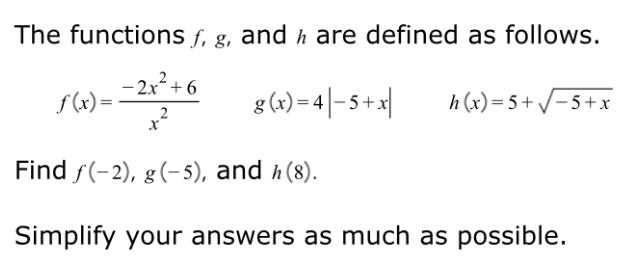# The functions f, g, and h are defined as follows.- 2x² + 6f(x) =g(x) = 4|- + x|h (x) = 5+ /-5+xFind f(-2), g(- 5), and h(8).Simplify your answers as much as possible.

Question
6 viewshelp_outlineImage TranscriptioncloseThe functions f, g, and h are defined as follows. - 2x² + 6 f(x) = g(x) = 4|- + x| h (x) = 5+ /-5+x Find f(-2), g(- 5), and h(8). Simplify your answers as much as possible. fullscreen
check_circle

star
star
star
star
star
1 Rating
Step 1

Substitute the value of x and determine the value of function.

Step 2

Substitute x=-2 in the function f(x)

Step 3

Substitute x=-5 in the...

### Want to see the full answer?

See Solution

#### Want to see this answer and more?

Solutions are written by subject experts who are available 24/7. Questions are typically answered within 1 hour.*

See Solution
*Response times may vary by subject and question.
Tagged in

### Math